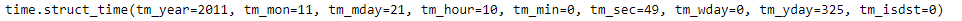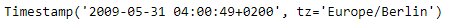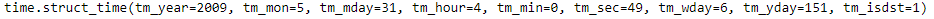# Python | Pandas Timestamp.timetuple

• Last Updated : 17 Jan, 2019

Python is a great language for doing data analysis, primarily because of the fantastic ecosystem of data-centric python packages. Pandas is one of those packages and makes importing and analyzing data much easier.

Pandas` Timestamp.timetuple()` function return a time tuple for the given Timestamp object. The returned tuple contains values ranging from year, month to hours and seconds. The time tuple is compatible with time.localtime().

Attention geek! Strengthen your foundations with the Python Programming Foundation Course and learn the basics.

To begin with, your interview preparations Enhance your Data Structures concepts with the Python DS Course. And to begin with your Machine Learning Journey, join the Machine Learning - Basic Level Course

Syntax :Timestamp.timetuple()

Parameters : None

Return : time tuple

Example #1: Use `Timestamp.timetuple()` function to return a time tuple for the given Timestamp object.

 `# importing pandas as pd``import` `pandas as pd`` ` `# Create the Timestamp object``ts ``=` `pd.Timestamp(year ``=` `2011``,  month ``=` `11``, day ``=` `21``, ``                  ``hour ``=` `10``, second ``=` `49``, tz ``=` `'US/Central'``) `` ` `# Print the Timestamp object``print``(ts)`

Output :Now we will use the `Timestamp.timetuple()` function to return a time tuple.

 `# return time tuple``ts.timetuple()`

Output :As we can see in the output, the `Timestamp.timetuple()` function has returned a tuple for the given Timestamp object which contains values like year, month, day etc.

Example #2: Use `Timestamp.timetuple()` function to return a time tuple for the given Timestamp object.

 `# importing pandas as pd``import` `pandas as pd`` ` `# Create the Timestamp object``ts ``=` `pd.Timestamp(year ``=` `2009``, month ``=` `5``, day ``=` `31``, ``                  ``hour ``=` `4``, second ``=` `49``, tz ``=` `'Europe/Berlin'``)`` ` `# Print the Timestamp object``print``(ts)`

Output :Now we will use the `Timestamp.timetuple()` function to return a time tuple.

 `# return time tuple``ts.timetuple()`

Output :As we can see in the output, the `Timestamp.timetuple()` function has returned a tuple for the given Timestamp object which contains values like year, month, day etc.

My Personal Notes arrow_drop_up